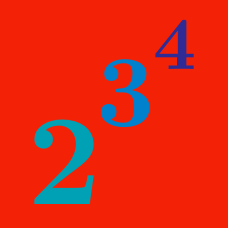Algebra

Rules of Exponents - Algebraic

If $3 ^ N = 3 ^ 5 \times 3 ^ 4$, what is the value of $N$?

What value of $N$ satisfies the equation

$\frac { 8 ^ {22}} { 8 ^ {11}} = 8 ^ N?$

Given $3^N = 9 ^{10}$, what is the value of $N$?

Hint: Rewrite 9 as $3^2.$

If $x$ is a real number satisfying $14 ^x = 3$, what is the value of $14 ^{2x + 1} ?$

$x$ and $y$ satisfy $2^x = 10$ and $10 ^ y = 128$. What is the value of $xy$?

Hint: Rewrite the "10" in $10^y = 128$ by using the fact that $2^x = 10.$

×